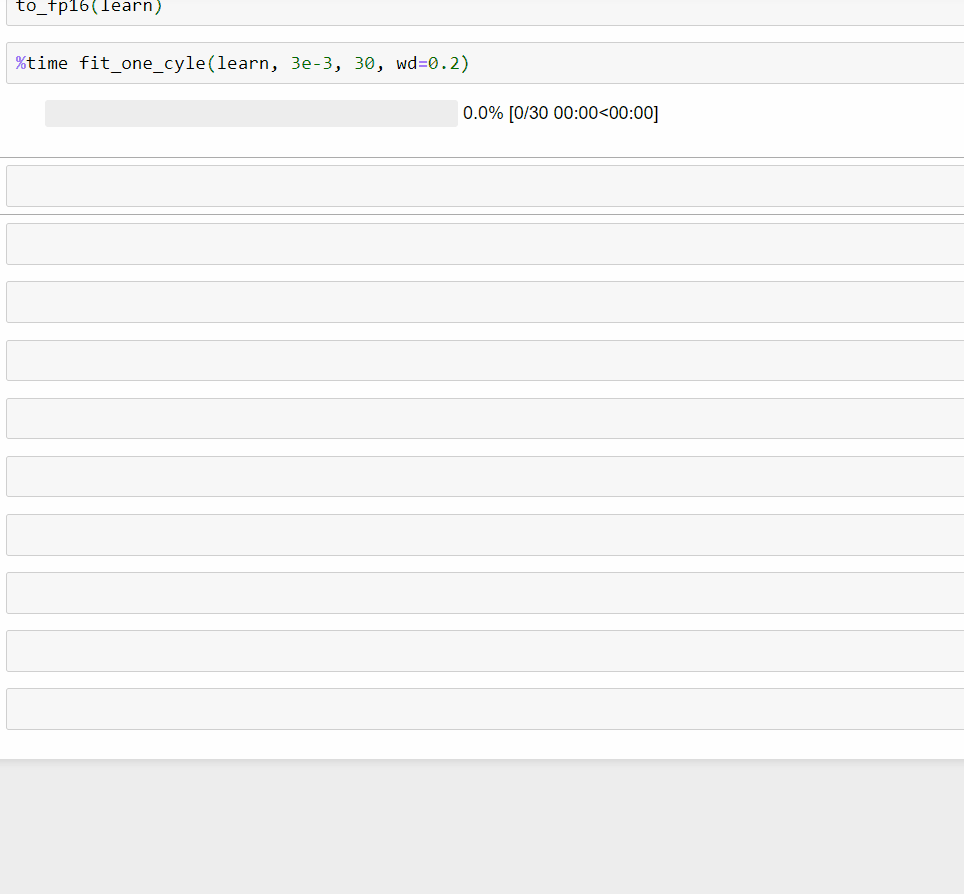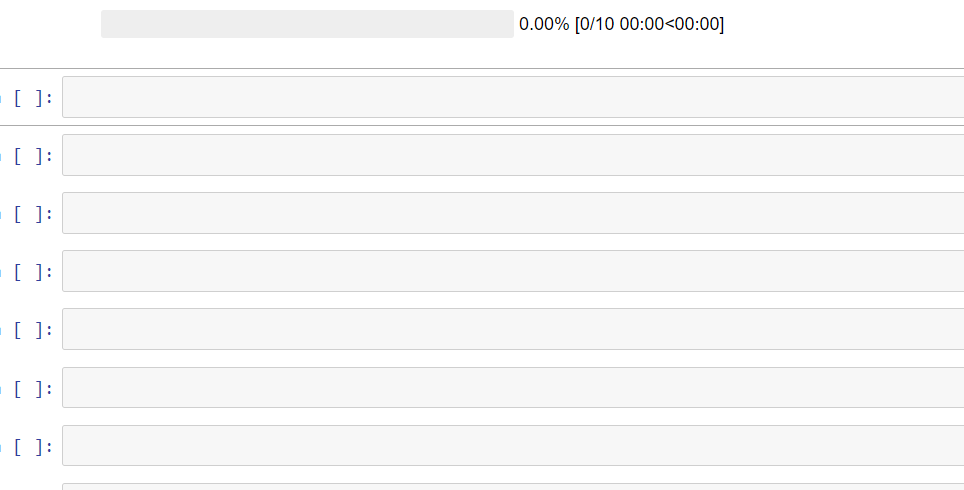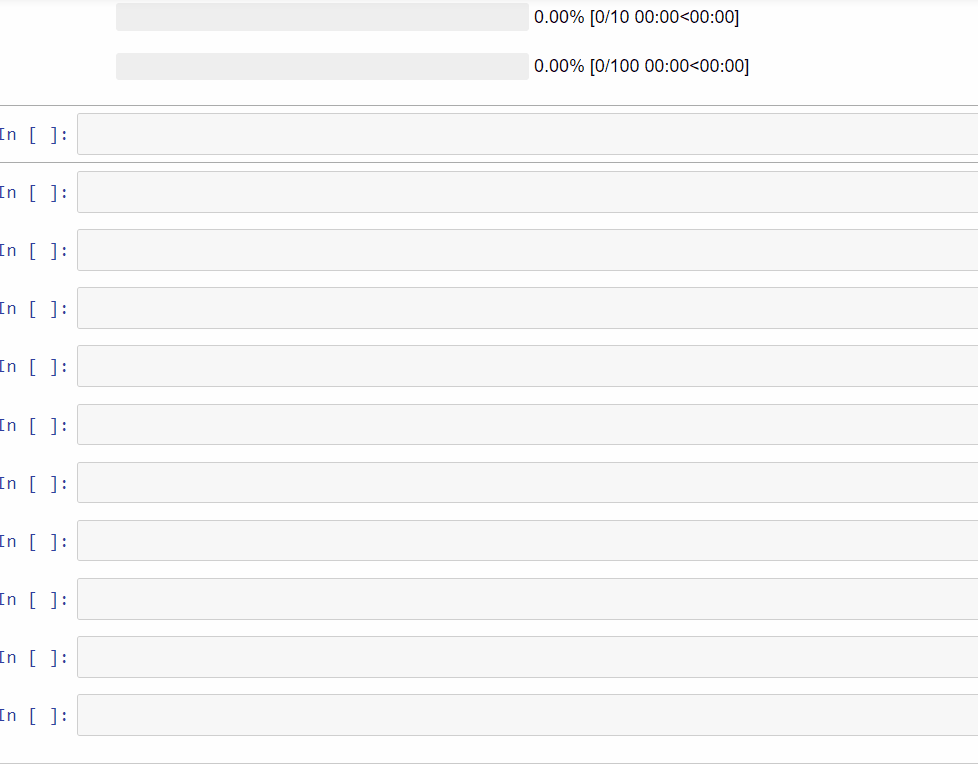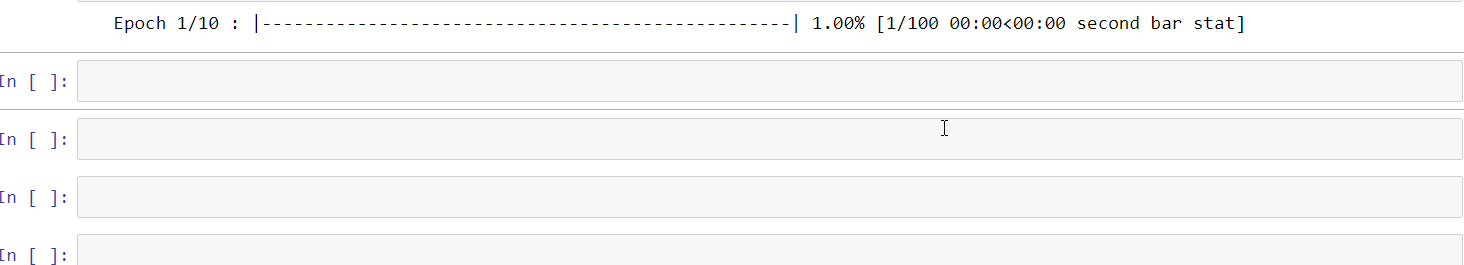A nested progress with plotting options for fastai

# fastprogress

A fast and simple progress bar for Jupyter Notebook and console. Created by Sylvain Gugger for fast.ai.

Copyright 2017 onwards, fast.ai. Licensed under the Apache License, Version 2.0 (the "License"); you may not use this file except in compliance with the License. A copy of the License is provided in the LICENSE file in this repository.## Install

To install simply use

pip install fastprogress


or:

conda install -c fastai fastprogress


Note that this requires python 3.6 or later.

## Usage

Here is a simple example. Each bar takes an iterator as a main argument, and we can specify the second bar is nested with the first by adding the argument parent=mb. We can then

• add a comment in the first bar by changing the value of mb.first_bar.comment
• add a comment in the first bar by changing the value of mb.child.comment
• write a line between the two bars with mb.write('message')
from fastprogress import master_bar, progress_bar
from time import sleep
mb = master_bar(range(10))
for i in mb:
for j in progress_bar(range(100), parent=mb):
sleep(0.01)
mb.child.comment = f'second bar stat'
mb.first_bar.comment = f'first bar stat'
mb.write(f'Finished loop {i}.')
#mb.update_graph(graphs, x_bounds, y_bounds)To add a graph that get plots as the training goes, just use the command mb.update_graphs. It will create the figure on its first use. Arguments are:

• graphs: a list of graphs to be plotted (each of the form [x,y])
• x_bounds: the min and max values of the x axis (if None, it will those given by the graphs)
• y_bounds: the min and max values of the y axis (if None, it will those given by the graphs)

Note that it's best to specify x_bounds and _bounds otherwise the box will change as the loop progresses.

Additionally, we can give the label of each graph via the command mb.names (should have as many elements as the graphs argument).

import numpy as np
mb = master_bar(range(10))
mb.names = ['cos', 'sin']
for i in mb:
for j in progress_bar(range(100), parent=mb):
if j%10 == 0:
k = 100 * i + j
x = np.arange(0, 2*k*np.pi/1000, 0.01)
y1, y2 = np.cos(x), np.sin(x)
graphs = [[x,y1], [x,y2]]
x_bounds = [0, 2*np.pi]
y_bounds = [-1,1]
mb.update_graph(graphs, x_bounds, y_bounds)
mb.child.comment = f'second bar stat'
mb.first_bar.comment = f'first bar stat'
mb.write(f'Finished loop {i}.')Here is the rendering in console:If the script using this is executed with a redirect to a file, only the results of the .write method will be printed in that file.

## Project details1.0.31.0.21.0.11.0.00.2.60.2.50.2.40.2.30.2.20.2.10.2.00.1.220.1.210.1.200.1.190.1.180.1.170.1.160.1.150.1.13

This version0.1.120.1.100.1.90.1.80.1.70.1.60.1.5

Uploaded source
Uploaded py3